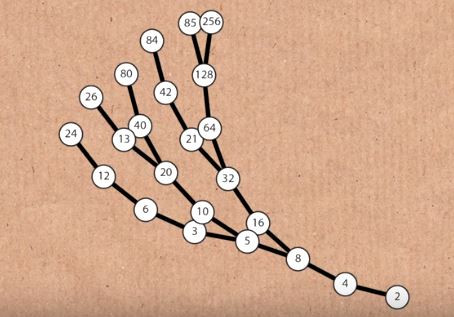Search

# The Collatz Conjecture and Other "Simple" Unsolved Problems

Updated: Nov 25, 2019

Try this.

Take any integer like 1, 2, 3, or 18.

If it is odd, multiply it by 3 and add 1.

If it is even, divide it by 2.

Stop when you notice a pattern.

Try another integer and another. Even try the first 20 or so.

What do you see?

Here, let me show you a few examples:

4 → 2 → 1

5 → 16 → 8 → 4 → 2 → 1

7 → 22 → 11 → 34 → 17 → 52 → 26 → 13 → 40 → 20 → 10 → 5 → 16 → 8 → 4 → 2 → 1

Strangely enough, all of these sequences end in 4 → 2 → 1, and 1*3 + 1 is 4 so these cycles begin to repeat forever:

5 → 16 → 8 → 4 → 2 → 1 → 4 → 2 → 1 → 4 → 2 → 1 ...

At this point, it might seem that we've accumulated enough evidence to say that every single sequence will end this way. In fact, mathematicians have used computers to test every integer from 1 to 87*2^60, which is an extremely large number, and they always come down to 1.

But mathematicians cannot fully establish that something is true without proving it, that is, establishing an argument that this will always remain true no matter what number we choose.

It is possible that 1 to 87*2^60 can all satisfy this principle, but 87*2^60 + 1 does not. Even something larger than a googol could defy this. (Scientists can make conclusions by accumulating large bodies of evidence; mathematicians do not, which is why their conclusions can hold for thousands of years because they're universally true.)

This problem is called the Collatz Conjecture as it was initially posed by Lothar Collatz in 1937. The challenge is to prove that every single positive integer will reach the 4 → 2 → 1 cycle at some point.

Visualizations of the Collatz Conjecture are surprisingly complex.

Here's one from the book Visions from the Universe:Essentially, this visual takes each path of the Collatz Conjecture into a tree format:Here, each step where you divide by 2 is represented by a vertical black line and each step where you multiply by 3 and 1 is represented by a red horizontal line.

Then, we tilt the tree to get a little more insight into how this works. The path goes slightly counterclockwise if the next number is odd and slightly clockwise if the next number is even if you view it from the bottom up. I also removed the red horizontal lines for the sake of simplicity.Finally, removing the numbers allows us to see the complexity of the problem in full light and to create the famous mathematical "seaweed" image we see above.

Watch Numberphile (https://www.youtube.com/watch?v=LqKpkdRRLZw) to see an in-depth discussion of how these visuals were created.

Here's one more visual, created with a similar but different concept:What's crazy about these visuals is just how complex they are. The fact that two very simple rules generate just complex figures blows my mind every time, and it gives us a glimpse of why this problem is so hard for mathematicians to solve.

Beyond the Collatz Conjecture, there are more of these simple but yet unsolved problems.

The Goldbach Conjecture states that every even integer can be written as the sum of two primes (where primes are integers whose only factors are 1 and itself).

The Twin Prime Conjecture states that there are an infinite number of prime numbers that are only two apart (such as 3 and 5, 5 and 7 and 11 and 13)

The Inscribed Square Theorem states that for any possible closed loop that doesn't cross itself, you can draw a square with all 4 corners on the loop, such as in the drawing below:All of these problems are simple to state, yet not even the smartest minds in the world have been able to solve them. It's for this reason these fields (here, number theory and geometry) are often called "elementary," not because they're easy to solve, but because it's often fairly easy to understand the problems as they are stated. Who knows, maybe one day you'll tackle one of these problems!